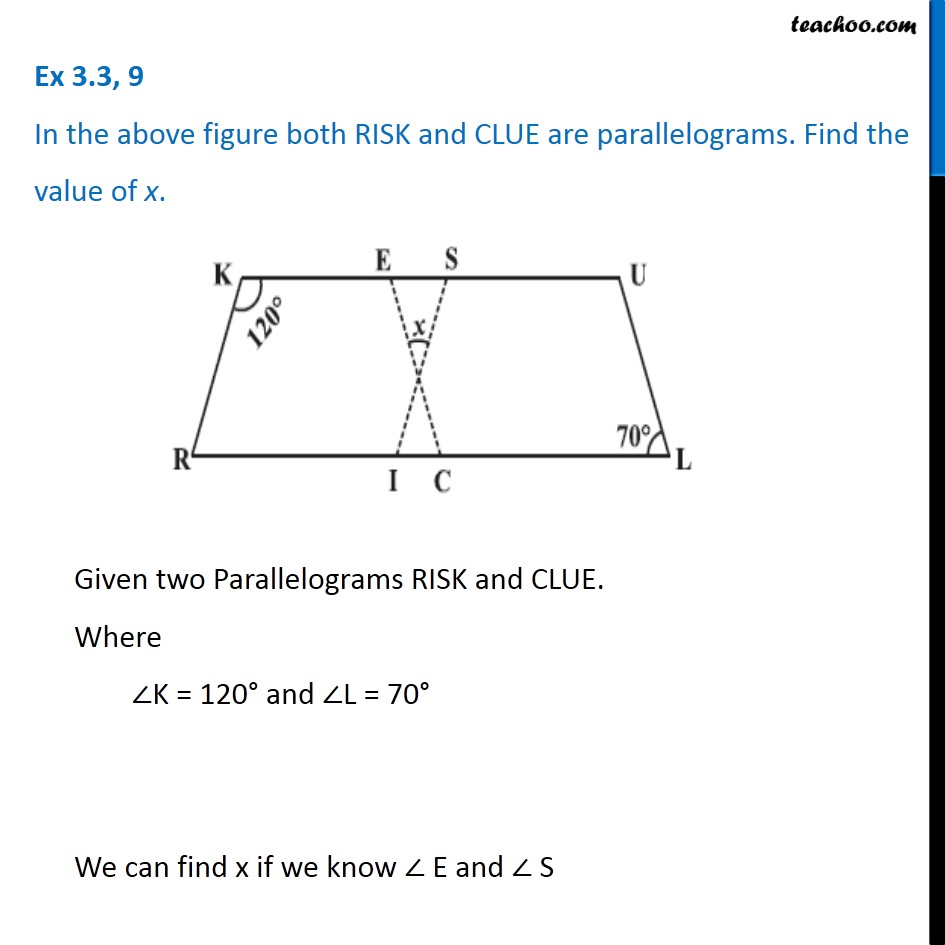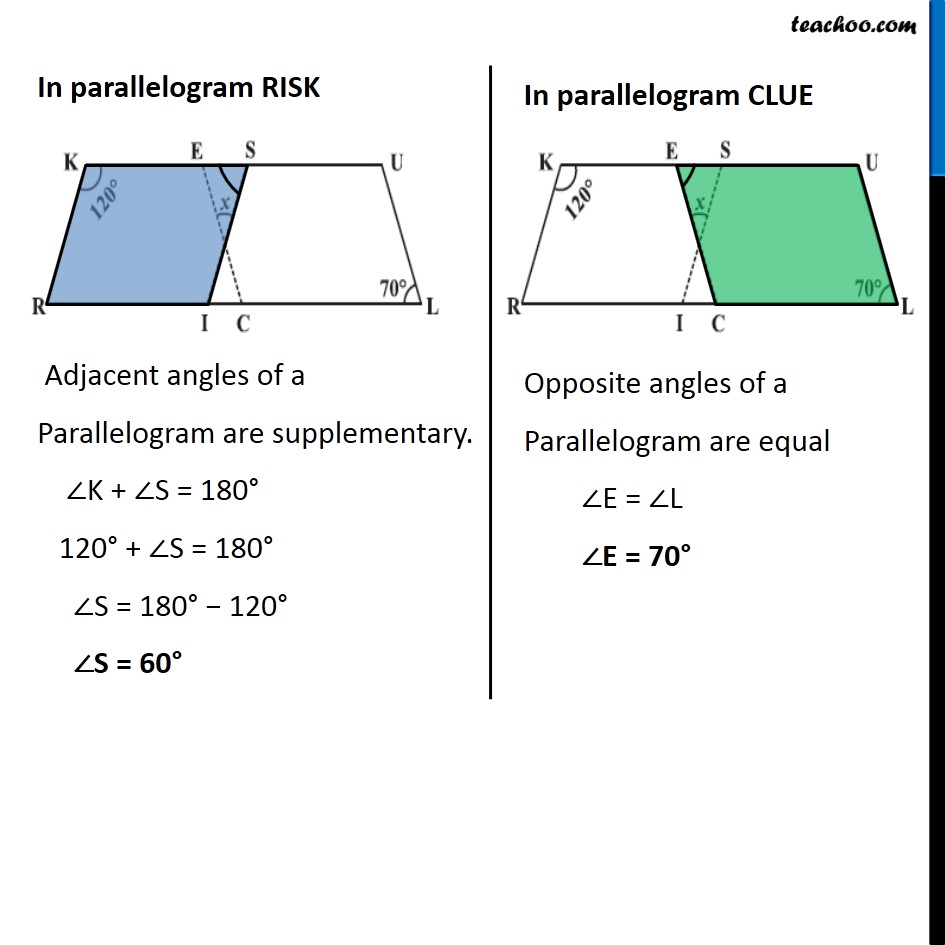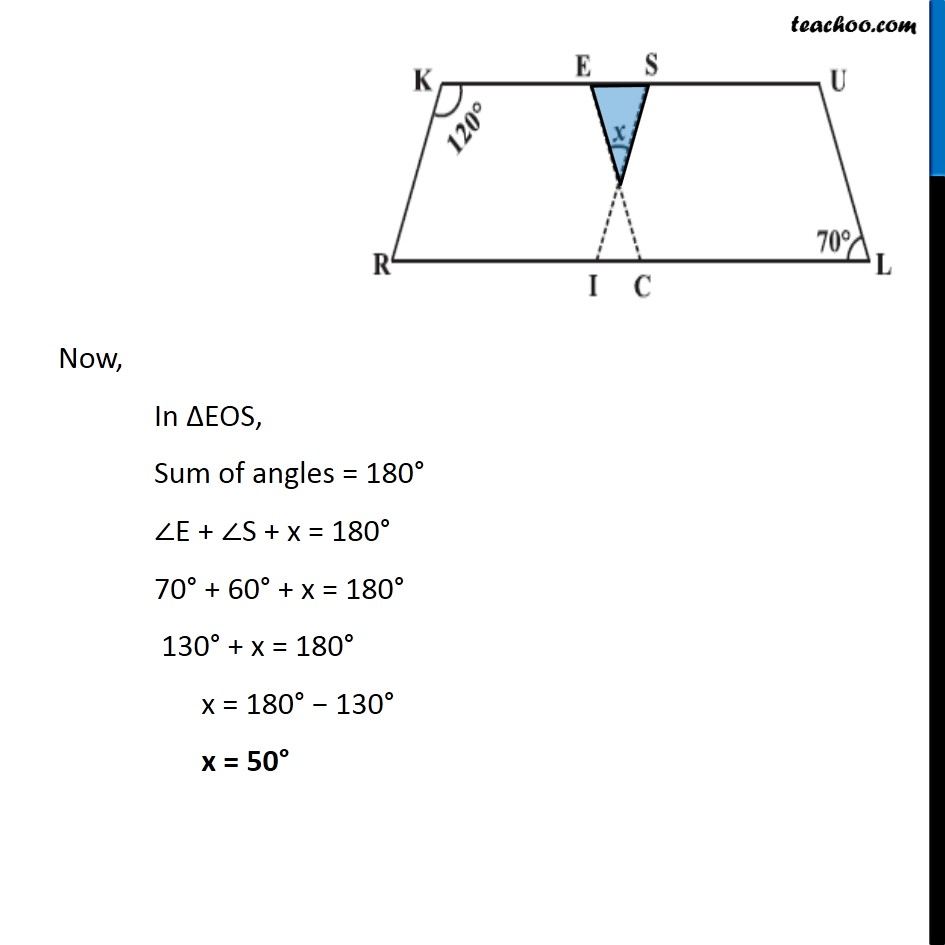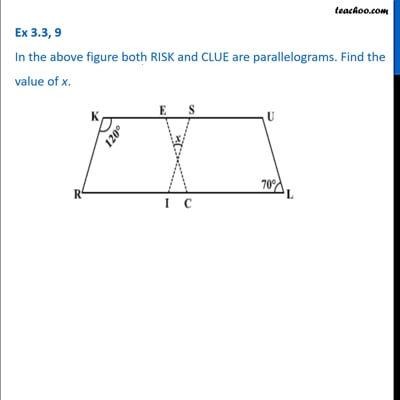Ex 3.3

Chapter 3 Class 8 Understanding Quadrilaterals
Serial order wiseThis video is only available for Teachoo black users

### Transcript

Ex 3.3, 9 In the above figure both RISK and CLUE are parallelograms. Find the value of x.Given two Parallelograms RISK and CLUE. Where ∠K = 120° and ∠L = 70° We can find x if we know ∠ E and ∠ S In parallelogram RISK Adjacent angles of a Parallelogram are supplementary. ∠K + ∠S = 180° 120° + ∠S = 180° ∠S = 180° − 120° ∠S = 60° In parallelogram CLUE Opposite angles of a Parallelogram are equal ∠E = ∠L ∠E = 70° Now, In ∆EOS, Sum of angles = 180° ∠E + ∠S + x = 180° 70° + 60° + x = 180° 130° + x = 180° x = 180° − 130° x = 50°